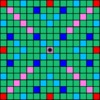# Scrabble scores (English)

Give the number of points each letter is worth in Scrabble. (The number of tiles bearing each letter is given as a clue.)

• Letter
• Points
• Frequency
• A
•
• x9
• B
•
• x2
• C
•
• x2
• D
•
• x4
• E
•
• x12
• F
•
• x2
• G
•
• x3
• H
•
• x2
• I
•
• x9
• J
•
• x1
• K
•
• x1
• L
•
• x4
• M
•
• x2
• N
•
• x6
• Letter
• Points
• Frequency
• O
•
• x8
• P
•
• x2
• Q
•
• x1
• R
•
• x6
• S
•
• x4
• T
•
• x6
• U
•
• x4
• V
•
• x2
• W
•
• x2
• X
•
• x1
• Y
•
• x2
• Z
•
• x1
• blank
•
• x2